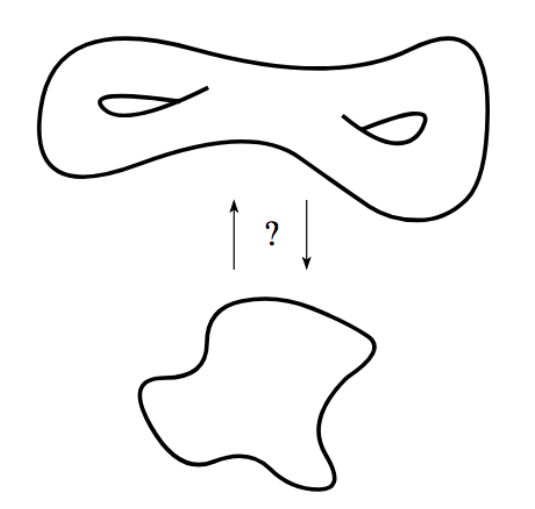# Explorations in Homeomorphic Variational Auto-Encoding

ICML 2018, Workshop on Theoretical Foundations and Applications of Deep Generative Models# Explorations in Homeomorphic Variational Auto-Encoding

ICML 2018, Workshop on Theoretical Foundations and Applications of Deep Generative Models### Abstract

The manifold hypothesis states that many kinds of high-dimensional data are concentrated near a low-dimensional manifold. If the topology of this data manifold is non-trivial, a continuous encoder network cannot embed it in a one-to-one manner without creating holes of low density in the latent space. This is at odds with the Gaussian prior assumption typically made in Variational Auto-Encoders (VAEs), because the density of a Gaussian concentrates near a blob-like manifold.

In this paper we investigate the use of manifold-valued latent variables. Specifically, we focus on the important case of continuously differentiable symmetry groups (Lie groups), such as the group of 3D rotations SO(3). We show how a VAE with SO(3)-valued latent variables can be constructed, by extending the reparameterization trick to compact connected Lie groups. Our experiments show that choosing manifold-valued latent variables that match the topology of the latent data manifold, is crucial to preserve the topological structure and learn a well-behaved latent space.

### Abstract

The manifold hypothesis states that many kinds of high-dimensional data are concentrated near a low-dimensional manifold. If the topology of this data manifold is non-trivial, a continuous encoder network cannot embed it in a one-to-one manner without creating holes of low density in the latent space. This is at odds with the Gaussian prior assumption typically made in Variational Auto-Encoders (VAEs), because the density of a Gaussian concentrates near a blob-like manifold.

In this paper we investigate the use of manifold-valued latent variables. Specifically, we focus on the important case of continuously differentiable symmetry groups (Lie groups), such as the group of 3D rotations SO(3). We show how a VAE with SO(3)-valued latent variables can be constructed, by extending the reparameterization trick to compact connected Lie groups. Our experiments show that choosing manifold-valued latent variables that match the topology of the latent data manifold, is crucial to preserve the topological structure and learn a well-behaved latent space.